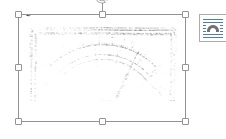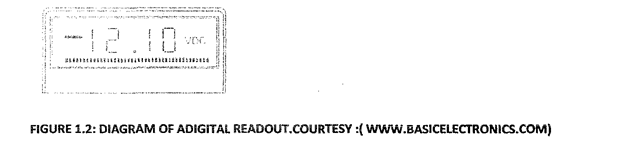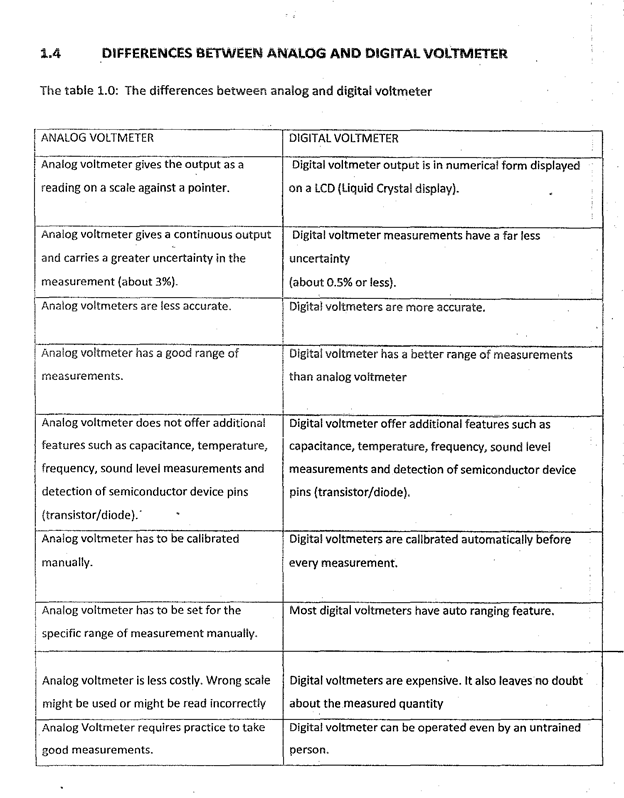# DESIGN AND CONSTRUCTION OF A DIGITAL VOLTMETER

## Content

ABSTRACT

This project is a digital voltmeter that uses the Integrating converter (ICL71O7) from INTERSIL. The lCL71O7 is a high performance, low power, 35 digit analog to digital converter The IC includes internal circuitry for seven segment decoders, display drivers, reference voltage source and a dock. The power dissipation is less than 10mW and the display stability is very high. The working of this electronic circuit is very simple. The voltage to be measured is converted into a dig equivalent by the analog to digital converter (A inside the IC and then this digital equivalent is decoded to the seven segment format and then displayed. The ADC used in 1CL7107 is a dual slope type ADC. The process taking place inside our ADC can be stated as follows. For a fixed period of time the voltage to be measured is integrated to obtain a ramp at the output of the integrator. Then a known reference voltage of opposite polarity is applied to the input of the integrator and allowed to ramp until the output of integrator becomes zero.

The problem that is usually encountered in using the analog display of voltmeter is that there is not much accuracy in the readings and the person using it has to calculate and interpret in hundred, kilo or mega volts. The analog voltmeter is displayed using a scale and a pointer, due to this, there is much parallax and human error in the readings which makes it less accurate.

The solution to the problem outlined above is to design a digital voltmeter which will eliminate parallax error and reduce human error and thereby giving high level of accuracy in the readings.

Another interesting thing about this digital voltmeter is that it uses LED (Light Emitting Diode) type, seven segment display and therefore can be seen clearly in darkness which most of analog voltmeter do not have.

Though the cost of producing a digital voltmeter is more than that of an analog type, but the advantage of a digital voltmeter is much more than of an analog type.

In consideration of the problems and solution suggested, it is concluded that a digital voltmeter is very useful and it will make life easier.

Title page

Certification page

Letter of submission

Dedication

Acknowledgement

Abstract

CHAPTER ONE

1.0     Introduction

1.1     Aims and objectives

1.2     Analog voltmeter

1.3     Digital voltmeter

1.4     Differences between analog and digital voltmeter

1.5     working operation of this project (methodology)

CHAPTER TWO

2.0     Literature review

2.1     Theory and operational principles of devices

2.2     Resistor

2.3     Capacitor

2.4     Analog to digital converter

2.5     Seven segment display

2.6     Potentiometer

2.7     differences between multi-meter and voltmeter

CHAPTER THREE

3.0     Design, Methodology and Operation

3.1     General description         25

3.2     Circuit diagram of a digital

3.3     Analog to digital converter ICL 7107

3.4     How the project works

3.5     Procedure for construction (design)

3.6     parts listed of components used

3.7     Challenges encountered and solution

3.8     Power supply

CHAPTER FOUR

4.0     Testing and conclusion

4.1     Measuring voltage

CHAPTER FIVE

5.0     Recommendation and observation

REFERENCES

CHAPTER ONE

1.0    INTRODUCTION

Electronic s system is a system that deals with the flow of electrons. Resistance, voltage and current are the basics of electronics. Electronics system refines, extends, supplements human facilities and gives us the ability to perceive, communicate, calculate, remember, reason and so on. General It makes life easier. Electronics device in modern days are now replacing electromechanical device. For example, an automated electronic switch that is programmed to operate at a specific time under a S condition makes a mechanical (manual) switch useless. Electronics system is preferable to mechanical system because it has a high degree of accuracy and efficiency.

Most electrical quantities are measured with a device called a meter. Voltage is measured with a voltmeter, current with an ammeter, resistance with an ohmmeter and power with a wattmeter. A meter that measures only one of the above quantities is cared a voltmeter. Voltmeters are often permanently connected (wired) into a circuit to provide continuous monitoring of an electrical quantity.

Electronics system is classified into two pan that is Analog and Digital electronics system. Analog system is a system that deals with discrete values of quantify and changes its output value linearly with the change in input value. Example is scale and a pointer device such as an analog multi-meter, a weight scale, analog voltmeter, analog clocks etc. A digital system is the one that produces only two output levels based on the range of values of the input that is its output is represented by two discrete levels only which is ‘1’ and ‘0’. This land values are called Sits taken from the word “Binary Digit”. The bits are used to perform many arithmetic and logical operations. The land 0 values are otherwise known as logic levels or logic states. The 0 level is called the LOW level while the 1 level is called the HIGH level. With this bit, it is easier for computer to process data and gives out the required output because it is only dealing with land 0 values. This makes it possible for me to design a digital voltmeter that will display values in form of figures instead of scale and pointer as to the case of an analog voltmeter.

The digital voltmeters are instruments that measure voltage or voltage drop in a circuit, They use solid-state components and display values digitally. Typically, d voltmeters are used to locate excessive resistance that may indicate an open circuit or ground. They are also used to identify low voltage or voltage drops that may indicate a poor connection.

Digital voltmeters are connected in parallel with the circuit being tested so that the meter can tap a small amount of current. The positive lead is connected to the circuits positive side and the negative lead is connected to the circuits ground. The digital voltmeters internal resistance is the input impedance, which is usually expressed in ohms per volt. This amount is relatively high in order to prevent the device from drawing significant current and disturbing the operation of the circuit being tested The sensitivity of the current meter and the value of the series resistance determine the range of voltages that dig voltmeters can measure.

Digital voltmeters are usually designed around a special type of analog-to-digital converter called an integrating converter. Voltmeter accuracy is affected by many factors, including temperature and supply voltage variations. They can measure a range of alternating current (Ac) voltages, direct current (DC voltages, or both AC and DC voltages. Devices typically display between three and seven digits. Some digital voltmeters can also capture minimum and maximum voltages called spike readings.

1.1    AIMS AND OBJECTIVE OF THIS PROJECT

The aim of this project is to design and construct a digital voltmeter using I 7107.

The objectives are as follows:

1. A digital voltmeter or DVM is used to take highly accurate voltage measurement and easy to read from distance.
2.  Digital voltmeter output is in numerical form displayed on an LED (light emitting diode).A digital voltmeter typically consists of an analog to digital converter (A/D) with a digital display, The analog signal is converted into a digital code proportionate to the magnitude of the signal.
3. Digital voltmeter measurements have a far less uncertainty (about 0 or less). The cost of constructing a digital voltmeter is low and few external components are used.
4. Digital voltmeter possesses some few components, small in size and easy to construct with simple adjustment.
5. A digital voltmeter mo has superior resolution and a higher order of accuracy with plus or minus 0.5%.it also indicates a negative quantity when polarity is reversed.

1.2    ANALOG VOLTMETER

An analog voltmeter uses the needle pointer to read out values. With this meter, the needle can point anywhere on the scale. When an analog meter is read, the reacting is generally taken to the nearest minor division mark. If the pointer is half way between marks, it is read as a half-division. Before reading a meter scale, you must figure out the value of each division of the scale. Look at the scale on the meter in figure 1.1; notice that there is a heavy line represents five units. Now count the numbers of divisions (called minor divisions) between 4 and 5 since there are five minor divisions between 4 and 5, each minor division has a value of 0.2 units. Therefore, each minor division on the scale represents 0 ampere, suppose the needle (pointer) on the meter in figure 1.1 is pointing to the second mark to the right of the 7 mark. The meter would be indicating 7.6 amperes.Figure 1.1: DIAGRAM OF AN ANALOG READOUT. COURTESY:( WWW.BASICELECTRONICS.COM)

1.3    DIGITAL VOLTMETER

The voltmeter shown in figure 1.2 eliminates the need to decide which mark is closest to the pointer. There is no guesswork in trying to decide whether the meter reading is 184.9 or 184.8.Digital voltmeters are usually specified by the number of digits in their readout When the most significant (left-most) digit can be only a 0 or a 1, it is counted as a ha 200-volt digital voltmeter in figure 1.2 is a three and half digit meter. Even though it is called a 200-volt meter it can measure a maximum of 199.9 volts.1.5    WORKING OPERATION OF THIS PROJECT (METHODOLOGY)

This digital voltmeter project is ideal to use for measuring the output voltage of a DC (Direct Current) power supply. It includes a 3.5-digit LED display with a negative voltage Indicator. The 1CL7107 is a high performance, low power, 3.5 digit analog to digital converter. The IC Includes internal circuitry for seven segment decoders, display drivers, reference voltage source and a clock. The power dissipation is less than 10mW and the display stability is very high.

It measures DC voltages from 0 to 199SV with a resolution of 0.IV. The voltmeter is based on single ICL71O7 chip and may be fitted on a small 3cm x 7cm printed circuit board. The circuit should be supplied with a by voltage supply and consumes only around 2SmA. 220 Ohm resistor should be connected to the PIN4 on the first LED (LIGHT EMITTING DIODE) display. The voltmeter can also be configured to measure different voltage ranges and Use 10K potentiometer to set the reference voltage between PIN 35 and PIN 36 of the ICL7Wq IC to display higher voltage resolution. P IM with 100K resistor will allow to measure 0- 19.9W voltages with 0.0W (l0mV) accuracy.

The working of this electronic circuit is very simple. The voltage to be measured is converted into a digital equivalent by the ADC inside the IC and then this digital equivalent is decoded to the seven segment format and then displayed. The ADC used in 1CL7107 is dual slope type ADC. The process taking place inside our AIX can be stated as follows,

1.   For a fixed period of time the voltage to be measured is integrated to obtain a ramp at the output of the integrator.

2.   Then a known reference voltage of opposite polarity is applied to the input of the integrator and allowed to ramp until the output of integrator becomes zero.

3.   The time taken for the negative slope to reach zero is measured in terms of the IC clock cycle and it will be proportional to the voltage under measurement.

4.   In simple words, the input voltage is compared to an internal reference voltage and the result is converted in a digital format.

The resistor R2 and C are used to set the frequency of IC Internal clock Capacitor C2 neutralizes the fluctuations in the internal reference voltage and increases the stability of the display.R4 controls the range of the voltmeter. Right most three displays are connected so that they can display all digits. The left most display is so connected that it can display only “1” and “-“. The pin 5 (representing the dot) is connected to ground only for the third display and its position needs to be changed when you change the range of the volt meter by altering R4. (R4=1.2K gives O-20V range, R4=IZK gives O-200V range).